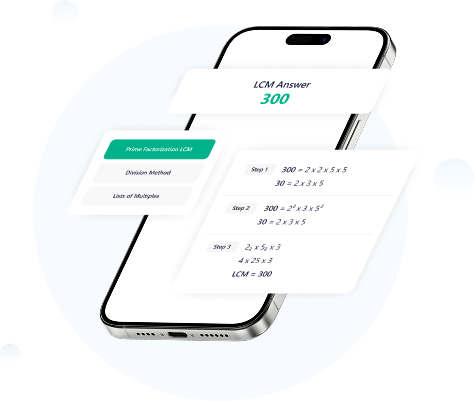# What is LCM and how to find it with different methods?

Publish Date:

If you are a student then you must be known about LCM as it is quite common but important as well.

LCM is not only used in the educational sector but it is also used for solving real-life problems like it can be used in many technologies.

Most of the students find it difficult to solve on their own but it is not that difficult, all you need is to have a proper basic concept.

Some methods are pretty common in mathematics and LCM is one of them. In contrast, there is another term named HCF so, don’t be confused in both of them.

We are going to take a start from the beginning so that you can surely make your concepts clearer about LCM.

And if you found solving LCM difficult then after reading this guide, you can surely solve your LCM problems on your own.

## What is LCM?

LCM stands for least common multiple and it must be known by many of the people. LCM is the smallest number that is the multiple of two given numbers.

We can say that if there are two given numbers and we have to find the LCM of both of these numbers then the LCM will be the smallest number that can be divisible by both of these numbers.

There is one thing to keep in mind and the thing is, LCM should always be a positive number.

## Is LCM a basic term?

It is not wrong to say that LCM can be the basis of many terms or mathematical problems and you will surely need LCM throughout your career or even your life.

As it is a base of many mathematical problems, we are going to discuss each and everything about the LCM, you can have a look.

## How to find LCM?

It is not that difficult to find the least common multiple, you just need the right and suitable method or you can use the LCM calculator so, let’s have a look.

There are some methods of finding LCM and these methods are pretty useful specifically when you are going to solve it on your own.

The methods are listed below, you can have a look at them.

• Listing multiples
• Prime factorization
• Division method

The above three methods that are listing multiples, division, and prime factors are pretty common and mostly used while finding LCM.

### 1. List of multiples

Suppose there are two numbers that are 12 and 18, if you are willing to solve the LCM using listing multiples then you need to find out the multiples of both of the numbers.

Multiples of 12: 12, 24, 36, 48, 60, 72…….

Multiples of 18: 18, 36, 54, 72……

Now, we need to take out the least common number between the multiples of both numbers.

Common numbers are 36 and 72, as we have mentioned above that LCM is the least number then 36 will be LCM of both of these numbers.

LCM of 12 and 18 = 36.

### 2. Prime factorization

Prime factorization is also a common method of finding an LCM and it is not wrong to say that it is also considered the easiest method.

So, let’s have a look,

We are going to take the LCM of 12 and 18 again so, first we have to take out the prime factors of both of the numbers.

Prime factors of 12: 2 × 2 × 3

Prime factors of 18: 2 × 3 × 3

To take out the LCM of these two numbers, we need to write them vertically but the matching digits must be vertically aligned.

2 × 2 × 3

2 × 3 × 3

Then you need to write all the prime factors in a single line and the final result will be:

2 × 2 × 3 × 3 = 36

So, the LCM of these two numbers is 36.

### 3. Division method

Suppose you are going to take the LCM of two numbers and the numbers are 20 and 30. You can simply write both of these numbers in a horizontal line.

Step 1: Divide these two numbers with the suitable prime number

Step 2: Take the product of prime numbers

The divisor numbers are 2x2x5x3, and then you can simply take the product of these numbers and that will be the least common multiple.

The final answer is 60 and this is the LCM of 20 and 30.

## Conclusion

When we talk about mathematical problems, there is always more than one way to solve them and the same goes for the least common multiple.

There are majorly three methods of taking LCM and we have discussed all these three methods above in detail.

It is not wrong to say that all these three methods are easy and useful so, you can go for any of them that can be much suitable for your mathematical problem.